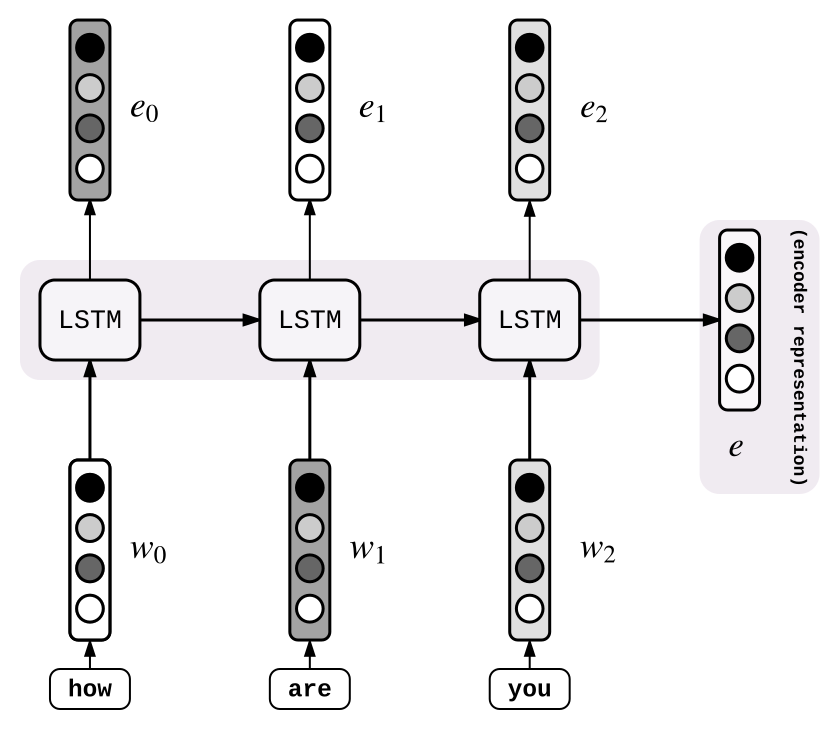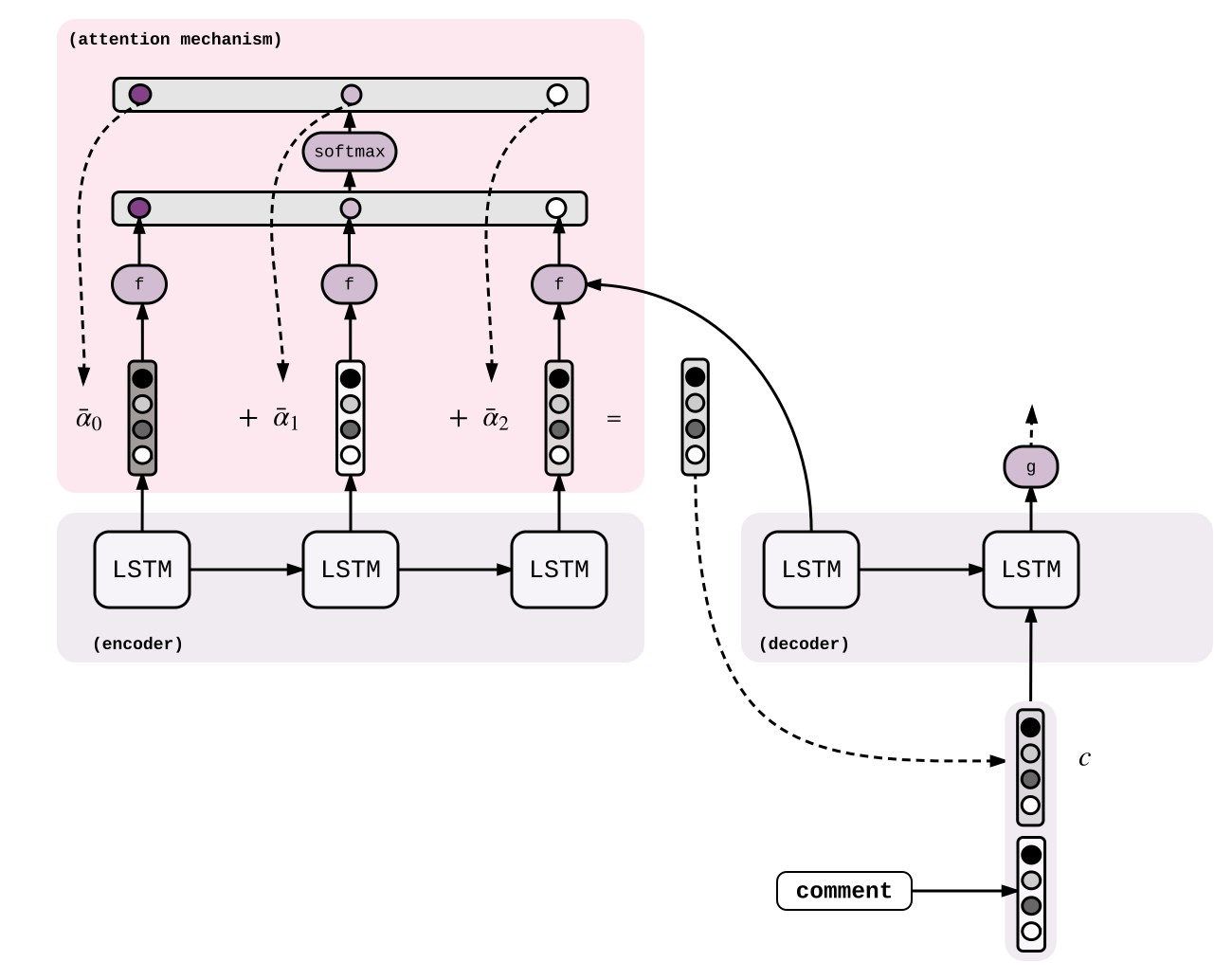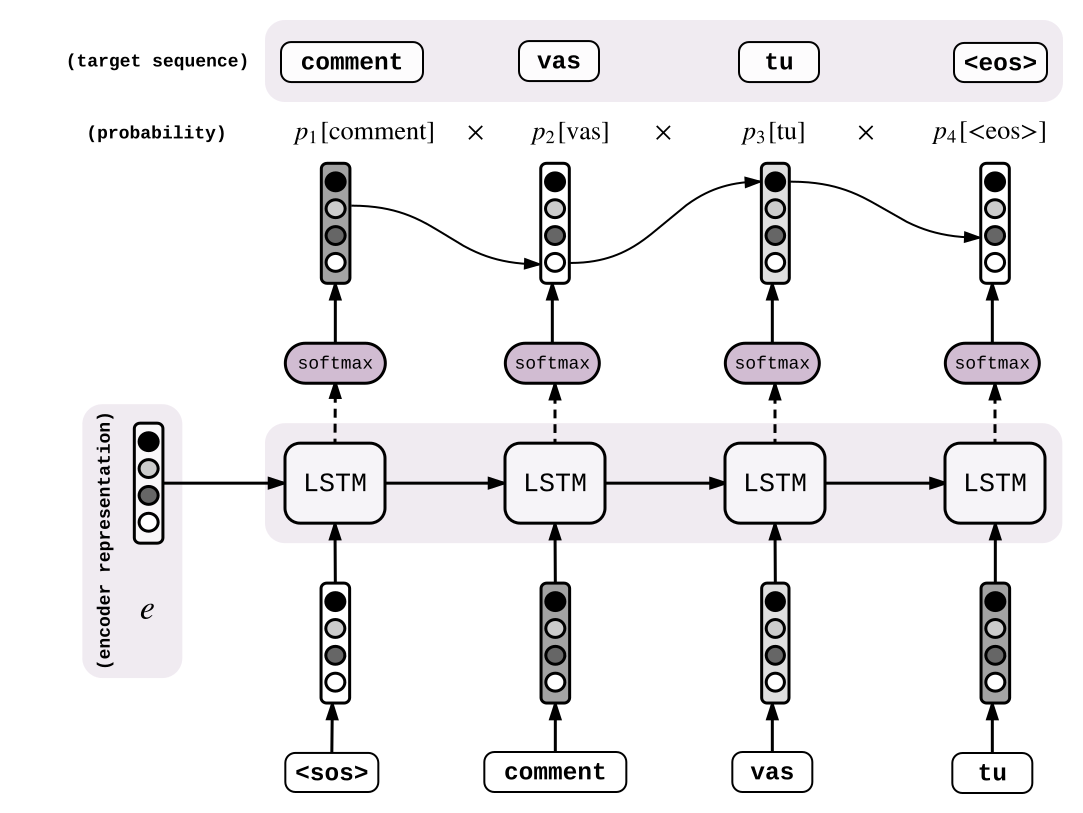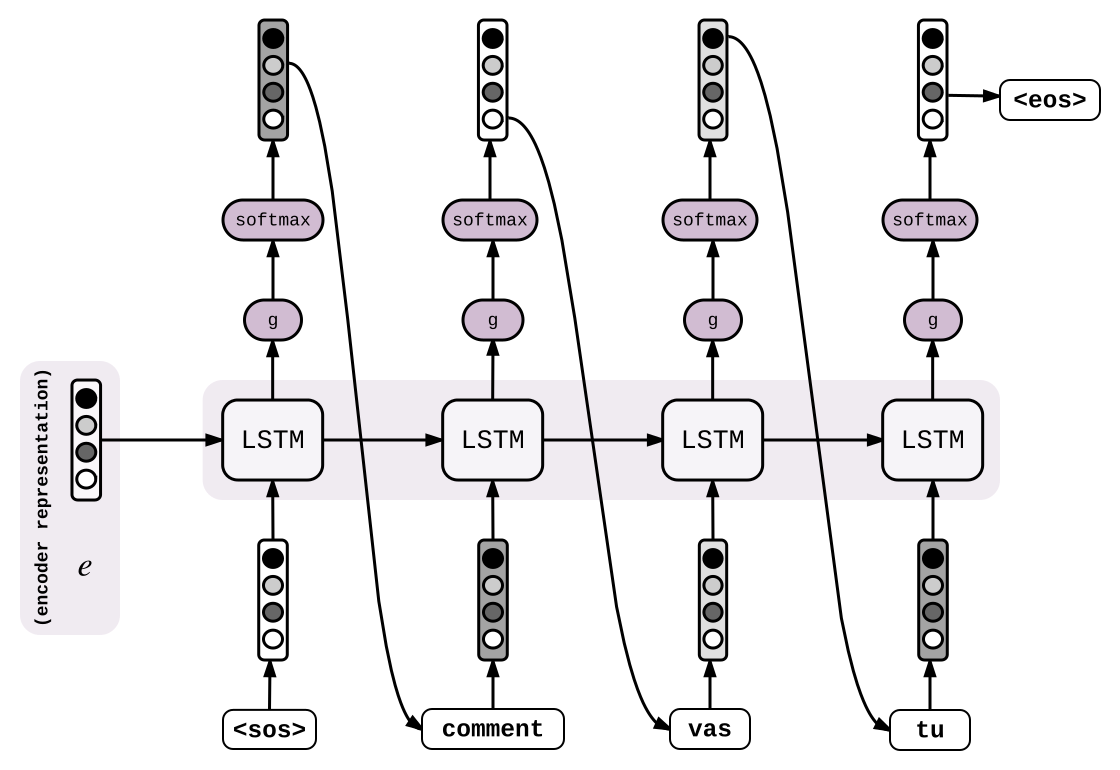2018年08月07日

## Sequence to Sequence 基本概念

### 普通Seq2Seq

Seq2Seq模型依赖于encoder-decoder结构。Encoder端对输入序列编码，然后Decoder端产生解码后的目标序列。

#### 编码器#### 解码器

$h_{0} = LSTM(e, w_{sos})$

,

$s_{0} = g(h{_0})$

,

$p_{0} = softmax(s_{0})$

,

$i_{0} = argmax(p_{0})$

$h_{1} = LSTM(h_{0}, w_{i_{0}})$

,

$s_{1} = g(h{_1})$

,

$p_{1} = softmax(s_{1})$

,

$i_{1} = argmax(p_{1})$

,

### 加入attention的Seq2Seq

,

$s_{t} = g(h_{t})$

,

$p_{t} = softmax(s_{t})$

,

$i_{t} = argmax(p_{t})$

,

,

$\overline{\alpha} = softmax(\alpha)$

,

$c_{t} = \sum_{t_{old}=0}^n\overline{\alpha}_{t_{old}}{e_{t_{old}}}$

,$f(h_{t-1},e_{t_{old}})=\begin{cases}h^{T}_{t-1}e_{t_{old}}&\text{dot}\\h^{T}_{t-1}We_{t_{old}}&\text{general}\\v^{T}tanh(W[h_{t-1},e_{t_{old}}])&\text{concat}\end{cases}$

,

#### 训练,

#### 解码### 结论

								 [看代码](https://anxingle.github.io)


Seq2Seq经典论文：

article translated from Guillaume Genthial Blog

-_-below can discuss!-_-